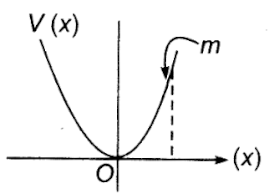A particle of mass m is released from rest and follows a parabolic path as shown in Fig. Assuming that the displacement of the mass from the origin is small, which graph correctly depicts the position of the particle as a function of time?

# A particle of mass m is released from rest and follows a parabolic path as shown in Fig. Assuming that the displacement of the mass from the origin is small, which graph correctly depicts the position of the particle as a function of time?1. A
2. B
3. C
4. D

Register to Get Free Mock Test and Study Material

+91

Verify OTP Code (required)

### Solution:

Restoring force
Equation of curve $\mathrm{y}={\mathrm{cx}}^{2}$

For small angle,
Since particle is executing SHM in a potential well after released from maximum displacement position.Register to Get Free Mock Test and Study Material

+91

Verify OTP Code (required)